## Chapter 12: Exercises

1. (**) 計算中文母音共振峰並畫圖: 請寫一個 MATLAB 程式 vowelFormantRecogChinese01.m，完成下列功能：
1. 進行五秒錄音，錄音規格是 16KHz/16Bits/Mono，錄音內容是中文母音「ㄚ、ㄧ、ㄨ、ㄝ、ㄛ」。（發音請盡量平穩，並在母音之間稍許停頓，以便後續進行自動切音，可以試聽此範例檔案。）
2. 使用 wave2formant.m (in ASR Toolbox) 來抓出兩個共振峰，相關規格是 formantNum=2, frameSize=20ms, frameStep=10ms, lpcOrder=12，這些參數也是 wave2formant.m 的預設參數。
3. 使用 endPointDetect.m (in SAP Toolbox) 來找出這五個音的開始和結束位置。請調整相關端點偵測參數，使得你的程式能夠自動地正確切出這五個音。你可以設定 plotOpt=1，以便使用 endPointDetect.m 來畫出端點偵測結果，正確圖形類似下圖：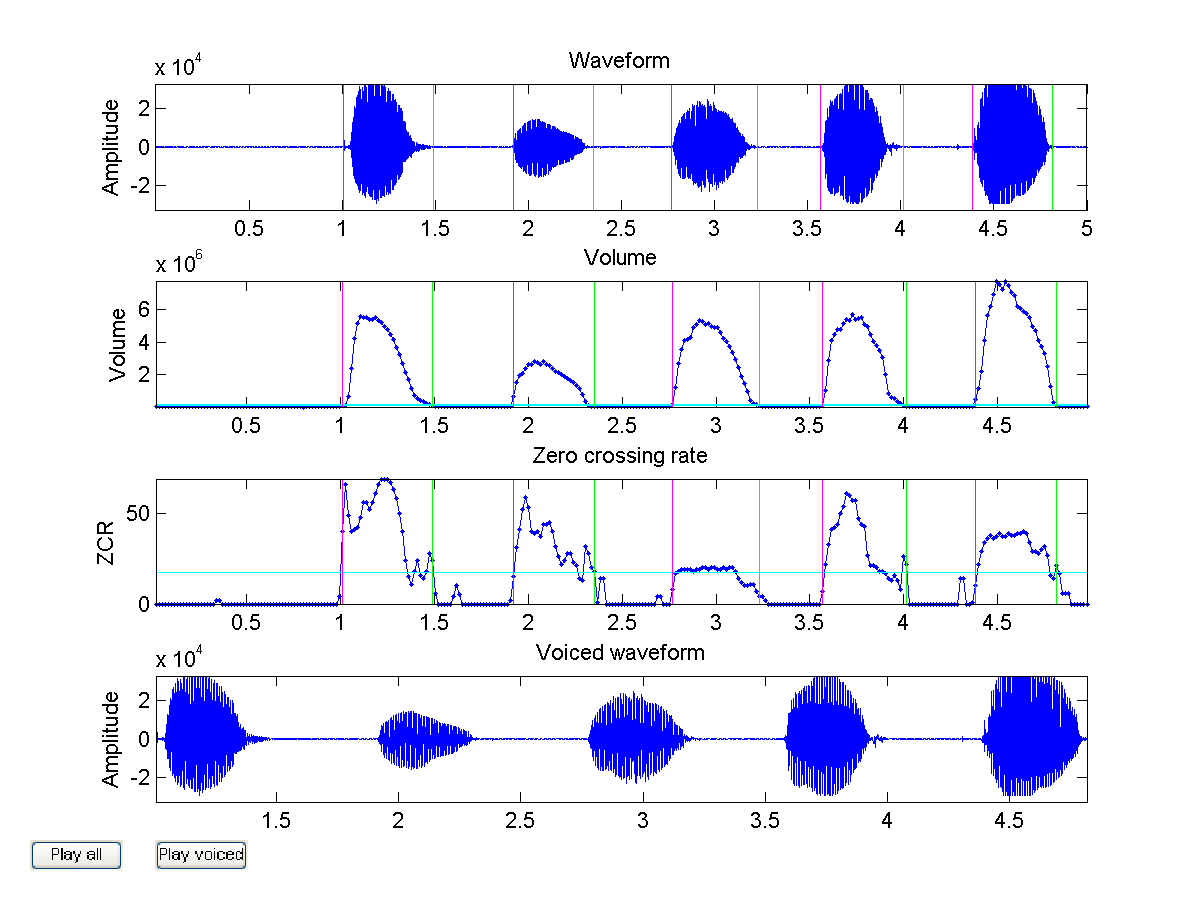4. 並用不同的顏色，在二度空間畫出這五個音的共振峰。畫出圖形應該類似下圖：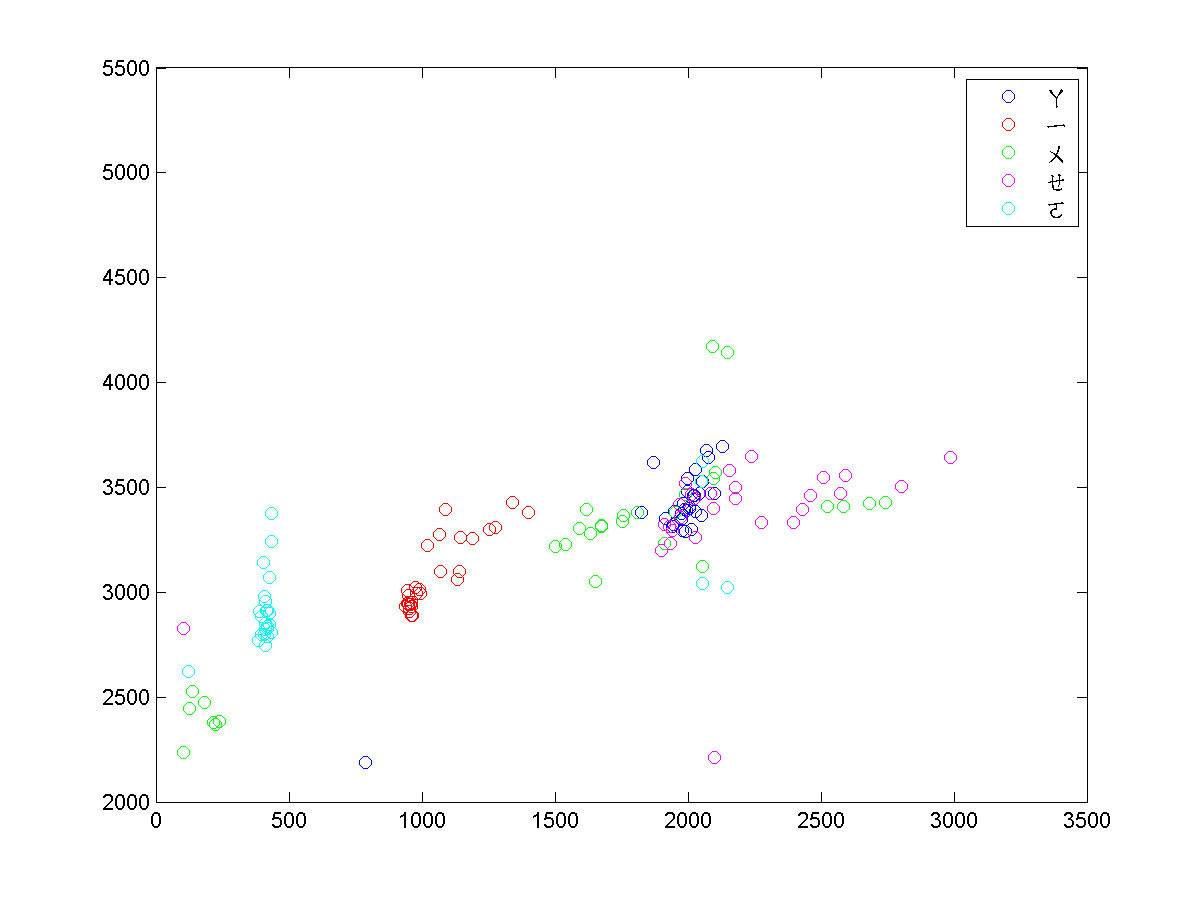5. 請用 knncLoo.m (in Machine Learning Toolbox) 來算出使用 knnc 將資料分成五類的 leave-one-out 辨識率。
2. (**) Frame to MFCC conversion: Write a MATLAB function frame2mfcc.m that can compute 12-dimensional MFCC from a given speech/audio frame. Please follow the steps in the text.
Solution: Pleae check out the function in the SAP Toolbox.
3. (***) Use MFCC for classifying vowels: Write an m-file script to do the following tasks:
1. Record a 5-second clips of the Chinese vowel 「ㄚ、ㄧ、ㄨ、ㄝ、ㄛ」 (or the English vowels "a, e, i, o, u") with 16KHz/16Bits/Mono. (Please try to maintain a stable pitch and volume, and keep a short pause between vowels to facilitate automatic vowel segmentation. Here is a sample file for your reference.)
2. Use epdByVol.m (in SAP Toolbox) to detect the starting and ending positions of these 5 vowels. If the segmentation is correct, you should have 5 sound segments from the 3rd output argument of epdByVol.m. Moreover, you should set plotOpt=1 to verify the segmentation result. Your plot should be similar to the following: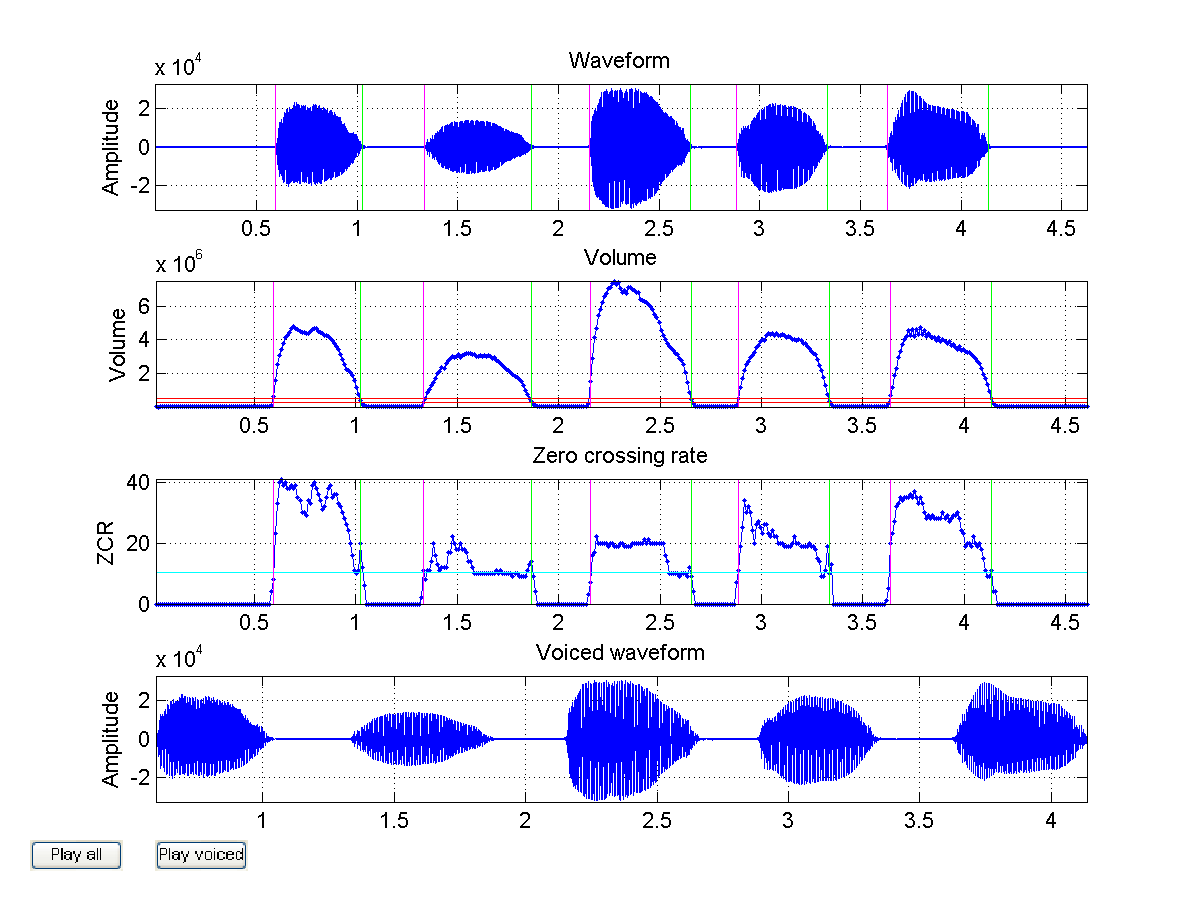If the segmentation is not correct, you should adjust the parameters to epdByVol.m until you get the correct segmentation.
3. Use buffer2.m to do frame blocking on these 5 vowels, with frameSize=32ms and overlap=0ms. Please generate 5 plots of MFCC (use frame2mfcc.m or wave2mfcc.m) corresponding to each vowel. Each plot should contains as many curves of MFCC vectors as the number of frames in this vowel. Your plots should be similar to those shown below: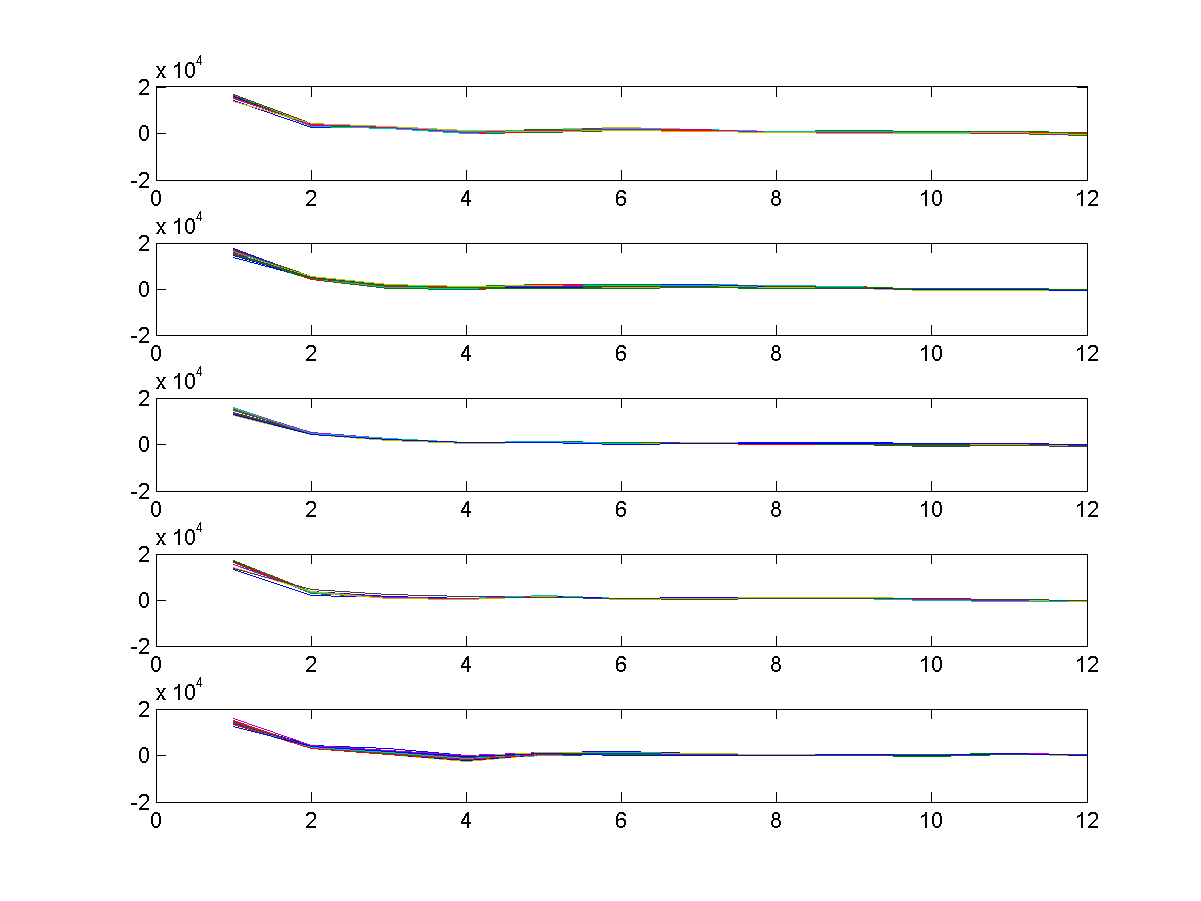4. Use knncLoo.m (in Machine Learning Toolbox) to compute the leave-one-our recognition rate when we use MFCC to classify each frame into 5 classes of different vowels. In particular, we need to change the dimension of the feature from 1 to 12 and plot the leave-one-out recognition rates using KNNC with k=1. What is the maximum recognition rate? What is the corresponding optimum dimension? Your plot should be similar to the next one: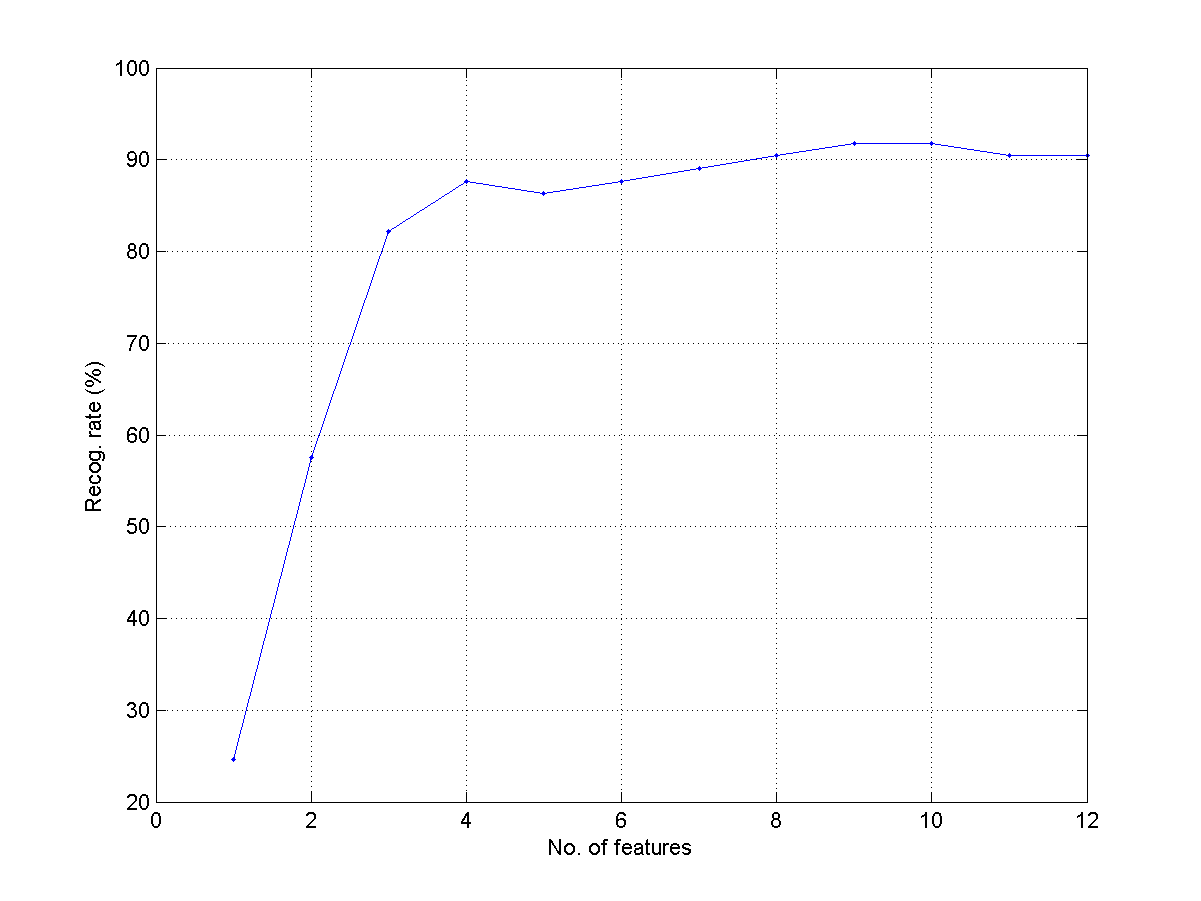5. Record another clip of the same utterance and use it as the test data. Use the original clip as the train data. Use the optimum dimension in the previous subproblem to compute the the frame-based recognition rate of KNNC with k=1. What is the frame-based recognition rate? Plot the confusion matrix, which should be similar to the following figure: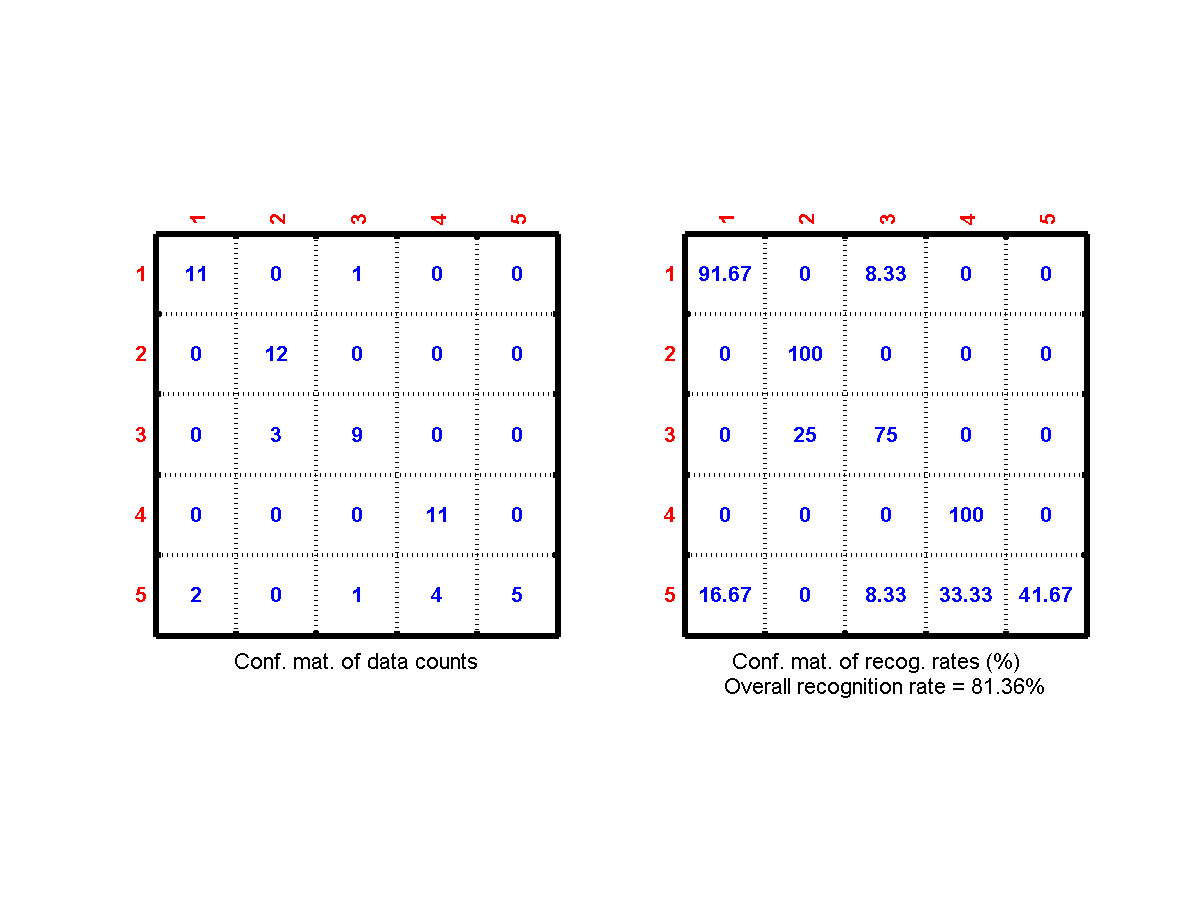6. What is the vowel-based recognition rate? Plot the confusion matrix, which should be similar to the following figure: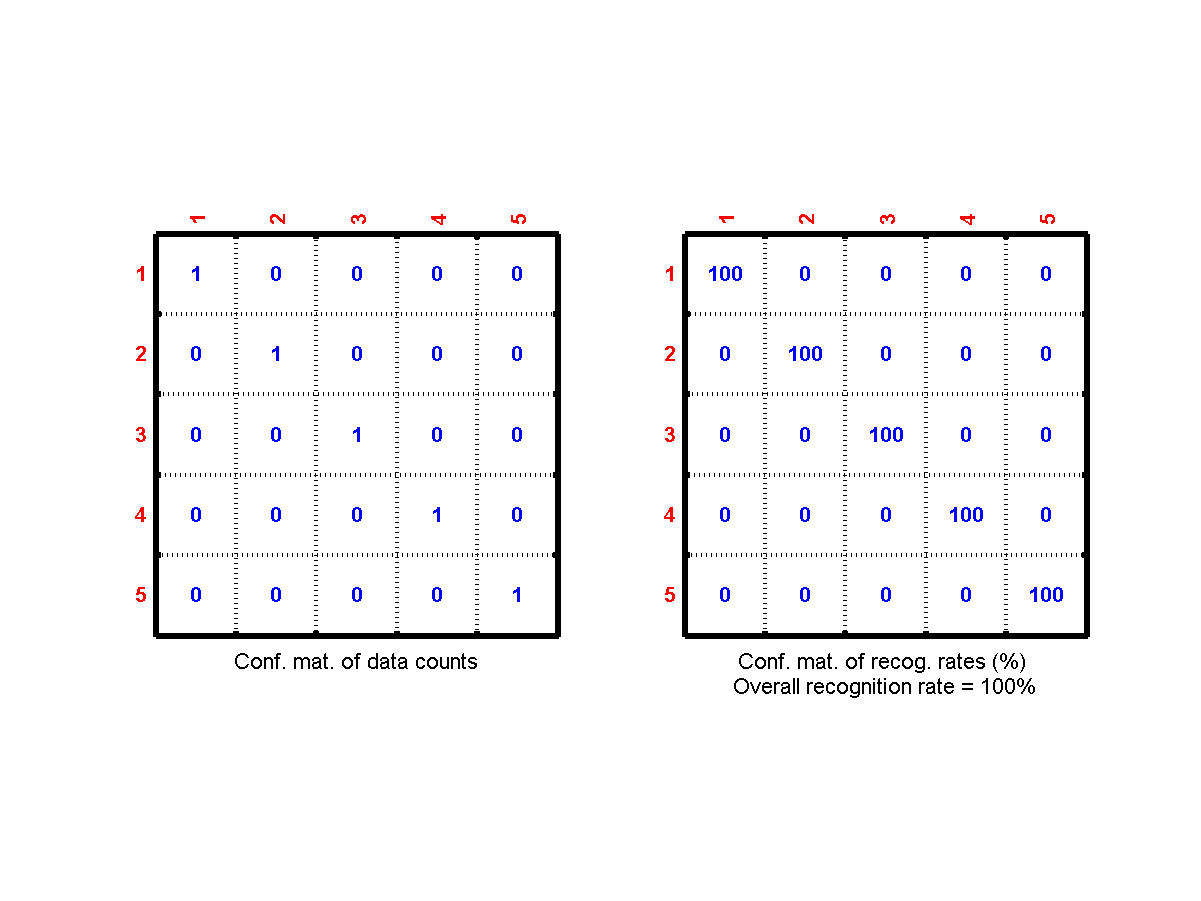(Hint: The vowel-based recognition result is the voting of frame-based results. You can use mode to compute the result of voting.)
7. Perform feature selection based on sequential forward selection to select up to 12 features. Plot the leave-one-out recognition rates with respective to the selected features. Your plot should be similar to the next one: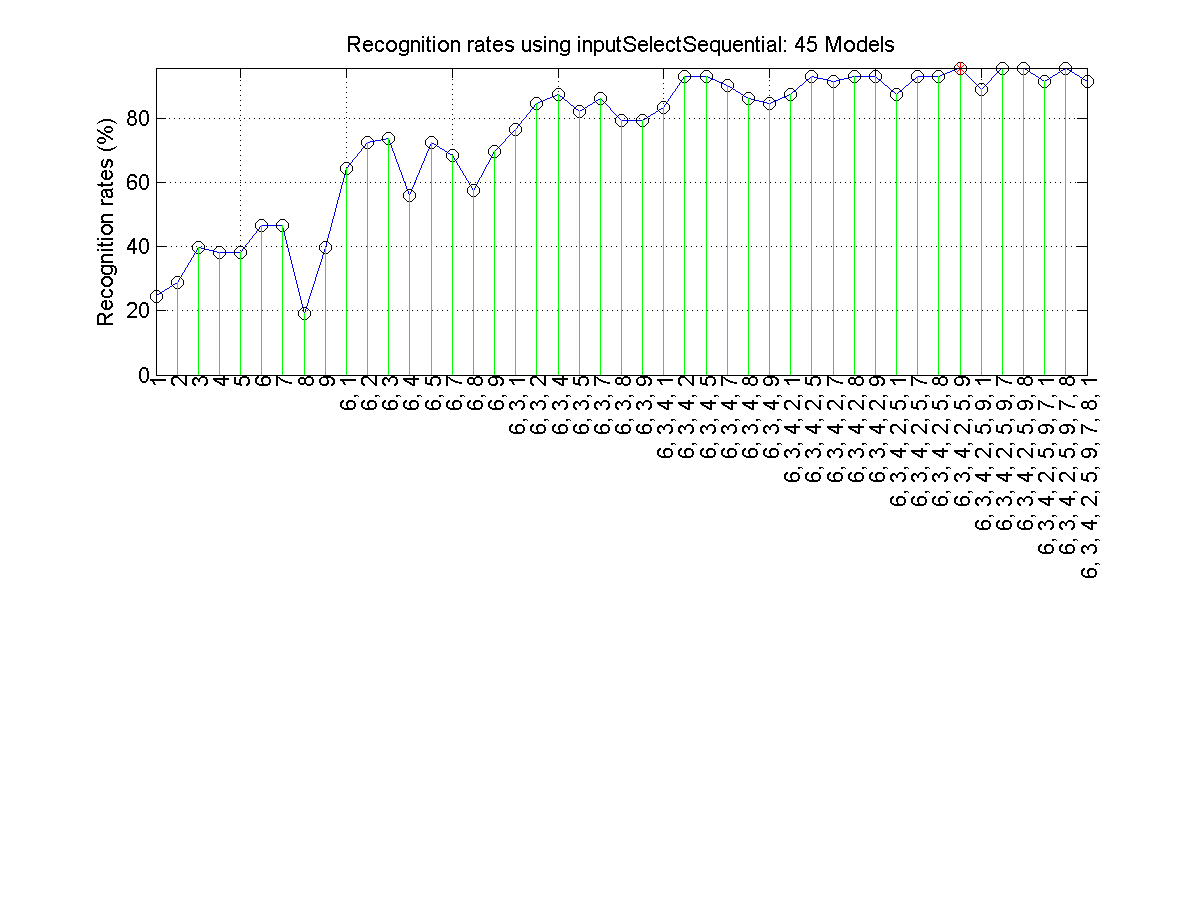What is the maximum recognition rate? What features are selected?
8. Use LDA to project 12-dimentional data onto 2D plane and plot the data to see if the data has the tendency of natural clustering based on their classes. Your plot should be similar to the next one: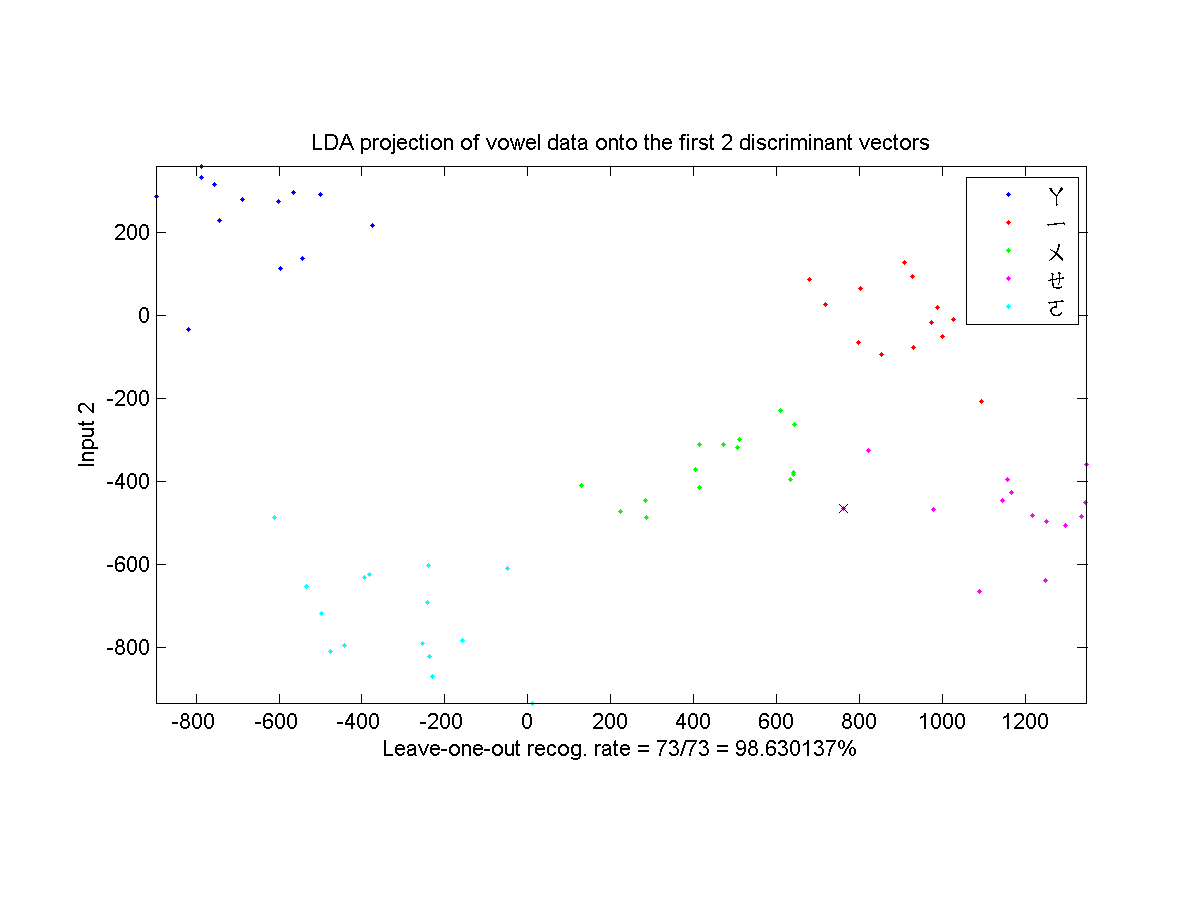What is the LOO recognition rate after LDA projection?
9. Repeat the previous sub-problem using PCA.

Audio Signal Processing and Recognition (音訊處理與辨識)